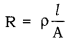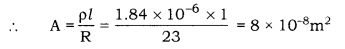# Calculate the area of cross section of a wire, if its length is 1.0m, its resistance is 23Ω

Calculate the area of cross section of a wire, if its length is 1.0m, its resistance is 23Ω and the resistivity of the material of the wire is 1.84 × 1O-6 Ωm.

We have,[where, R is a resistance]
Where, A is the area of cross-section of the wire
l = Length of the wire = 1 m
R = 23Ω
p = Resistivity of the wire = 1.84 × 10-6 ΩmTherefore, the area of cross-section of the wire is 8 × 10-8 m2.